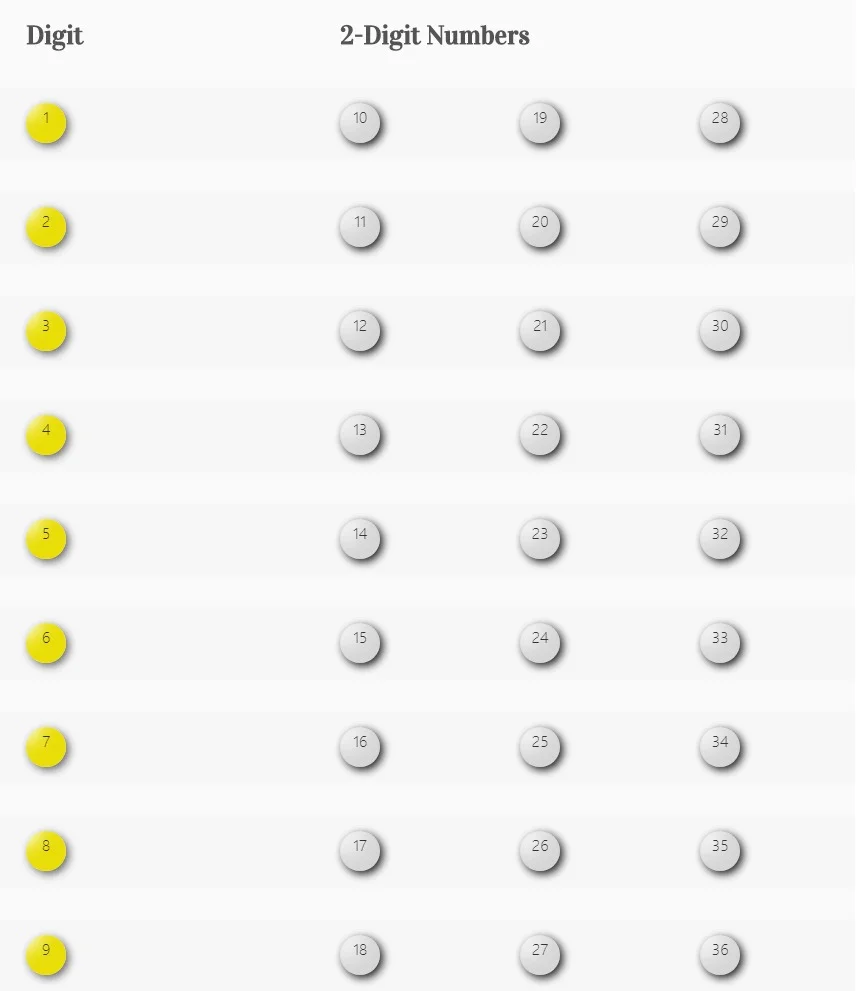## Nlcb Play Whe 1-10 Number Line Chart

In the Nlcb Play Whe 1-10 Number Line Chart, it is suggested that the digits 1 to 9 be played along with other associated two-digit numbers in a line based on the sum of the two digits in the number as shown in the chart below.

For example, the number 10 is associated with the digit 1 since adding the digits in the number 10 results in a sum of 1: 1+0=1. Adding the digits 2 and 8 in the number 28, or adding the digits 1 and 9 in the number 19 also gives us the sum of 10, so the number 1 will be associated with the numbers 10, 19 and 28.

To use the chart, players will often play the associated two-digit associated numbers along with any of the 1-digit numbers between 1 and 9, or they may add the two digits in numbers between 10 and 36 to also play corresponding 1-digit numbers.